moveit2 The MoveIt Motion Planning Framework for ROS 2.
robot_trajectory::RobotTrajectory Class Reference

Maintain a sequence of waypoints and the time durations between these waypoints. More...

`#include <robot_trajectory.h>`

class  Iterator

## Public Member Functions

RobotTrajectory (const moveit::core::RobotModelConstPtr &robot_model)
construct a trajectory for the whole robot More...

RobotTrajectory (const moveit::core::RobotModelConstPtr &robot_model, const std::string &group)
construct a trajectory for the named JointModelGroup If group is an empty string, this is equivalent to the first constructor, otherwise it is equivalent to `RobotTrajectory(robot_model, robot_model->getJointModelGroup(group))`. More...

RobotTrajectory (const moveit::core::RobotModelConstPtr &robot_model, const moveit::core::JointModelGroup *group)
construct a trajectory for the JointModelGroup Only joints from the specified group will be considered in this trajectory, even though all waypoints still consist of full RobotStates (representing all joints). More...

RobotTrajectoryoperator= (const RobotTrajectory &)=default

RobotTrajectory (const RobotTrajectory &other, bool deepcopy=false)
Copy constructor allowing a shallow or deep copy of waypoints. More...

const moveit::core::RobotModelConstPtr & getRobotModel () const

const moveit::core::JointModelGroupgetGroup () const

const std::string & getGroupName () const

RobotTrajectorysetGroupName (const std::string &group_name)

std::size_t getWayPointCount () const

std::size_t size () const

const moveit::core::RobotStategetWayPoint (std::size_t index) const

const moveit::core::RobotStategetLastWayPoint () const

const moveit::core::RobotStategetFirstWayPoint () const

moveit::core::RobotStatePtr & getWayPointPtr (std::size_t index)

moveit::core::RobotStatePtr & getLastWayPointPtr ()

moveit::core::RobotStatePtr & getFirstWayPointPtr ()

const std::deque< double > & getWayPointDurations () const

double getWayPointDurationFromStart (std::size_t index) const
Returns the duration after start that a waypoint will be reached. More...

double getWaypointDurationFromStart (std::size_t index) const

double getWayPointDurationFromPrevious (std::size_t index) const

RobotTrajectorysetWayPointDurationFromPrevious (std::size_t index, double value)

bool empty () const

RobotTrajectoryaddSuffixWayPoint (const moveit::core::RobotState &state, double dt)
Add a point to the trajectory. More...

RobotTrajectoryaddSuffixWayPoint (const moveit::core::RobotStatePtr &state, double dt)
Add a point to the trajectory. More...

RobotTrajectoryaddPrefixWayPoint (const moveit::core::RobotState &state, double dt)

RobotTrajectoryaddPrefixWayPoint (const moveit::core::RobotStatePtr &state, double dt)

RobotTrajectoryinsertWayPoint (std::size_t index, const moveit::core::RobotState &state, double dt)

RobotTrajectoryinsertWayPoint (std::size_t index, const moveit::core::RobotStatePtr &state, double dt)

RobotTrajectoryappend (const RobotTrajectory &source, double dt, size_t start_index=0, size_t end_index=std::numeric_limits< std::size_t >::max())
Add a specified part of a trajectory to the end of the current trajectory. The default (when `start_index` and `end_index` are omitted) is to add the whole trajectory. More...

void swap (robot_trajectory::RobotTrajectory &other)

RobotTrajectoryclear ()

double getDuration () const

double getAverageSegmentDuration () const

void getRobotTrajectoryMsg (moveit_msgs::msg::RobotTrajectory &trajectory, const std::vector< std::string > &joint_filter=std::vector< std::string >()) const

RobotTrajectorysetRobotTrajectoryMsg (const moveit::core::RobotState &reference_state, const trajectory_msgs::msg::JointTrajectory &trajectory)
Copy the content of the trajectory message into this class. The trajectory message itself is not required to contain the values for all joints. For this reason a full starting state must be specified as reference (reference_state). Each point in the trajectory to be constructed internally is obtained by copying the reference state and overwriting the content from a trajectory point in trajectory. More...

RobotTrajectorysetRobotTrajectoryMsg (const moveit::core::RobotState &reference_state, const moveit_msgs::msg::RobotTrajectory &trajectory)
Copy the content of the trajectory message into this class. The trajectory message itself is not required to contain the values for all joints. For this reason a full starting state must be specified as reference (reference_state). Each point in the trajectory to be constructed internally is obtained by copying the reference state and overwriting the content from a trajectory point in trajectory. More...

RobotTrajectorysetRobotTrajectoryMsg (const moveit::core::RobotState &reference_state, const moveit_msgs::msg::RobotState &state, const moveit_msgs::msg::RobotTrajectory &trajectory)
Copy the content of the trajectory message into this class. The trajectory message itself is not required to contain the values for all joints. For this reason a full starting state must be specified as reference (reference_state). Before use, the reference state is updated using state. Each point in the trajectory to be constructed internally is obtained by copying the reference state and overwriting the content from a trajectory point in trajectory. More...

RobotTrajectoryreverse ()

RobotTrajectoryunwind ()

RobotTrajectoryunwind (const moveit::core::RobotState &state)
Unwind, starting from an initial state. More...

void findWayPointIndicesForDurationAfterStart (const double &duration, int &before, int &after, double &blend) const
Finds the waypoint indices before and after a duration from start. More...

bool getStateAtDurationFromStart (const double request_duration, moveit::core::RobotStatePtr &output_state) const
Gets a robot state corresponding to a supplied duration from start for the trajectory, using linear time interpolation. More...

RobotTrajectory::Iterator begin ()

RobotTrajectory::Iterator end ()

void print (std::ostream &out, std::vector< int > variable_indexes=std::vector< int >()) const
Print information about the trajectory. More...

## Detailed Description

Maintain a sequence of waypoints and the time durations between these waypoints.

Definition at line 59 of file robot_trajectory.h.

## ◆ RobotTrajectory() [1/4]

 robot_trajectory::RobotTrajectory::RobotTrajectory ( const moveit::core::RobotModelConstPtr & robot_model )
explicit

construct a trajectory for the whole robot

Definition at line 50 of file robot_trajectory.cpp.

## ◆ RobotTrajectory() [2/4]

 robot_trajectory::RobotTrajectory::RobotTrajectory ( const moveit::core::RobotModelConstPtr & robot_model, const std::string & group )

construct a trajectory for the named JointModelGroup If group is an empty string, this is equivalent to the first constructor, otherwise it is equivalent to `RobotTrajectory(robot_model, robot_model->getJointModelGroup(group))`.

Definition at line 55 of file robot_trajectory.cpp.

## ◆ RobotTrajectory() [3/4]

 robot_trajectory::RobotTrajectory::RobotTrajectory ( const moveit::core::RobotModelConstPtr & robot_model, const moveit::core::JointModelGroup * group )

construct a trajectory for the JointModelGroup Only joints from the specified group will be considered in this trajectory, even though all waypoints still consist of full RobotStates (representing all joints).

If group is nullptr this is equivalent to the first constructor.

Definition at line 60 of file robot_trajectory.cpp.

## ◆ RobotTrajectory() [4/4]

 robot_trajectory::RobotTrajectory::RobotTrajectory ( const RobotTrajectory & other, bool deepcopy = `false` )

Copy constructor allowing a shallow or deep copy of waypoints.

Parameters
 other - RobotTrajectory to copy from deepcopy - copy waypoints by value (true) or by pointer (false)?

Definition at line 66 of file robot_trajectory.cpp.

## Member Function Documentation

 RobotTrajectory& robot_trajectory::RobotTrajectory::addPrefixWayPoint ( const moveit::core::RobotState & state, double dt )
inline

Definition at line 208 of file robot_trajectory.h.

Here is the caller graph for this function:RobotTrajectory& robot_trajectory::RobotTrajectory::addPrefixWayPoint ( const moveit::core::RobotStatePtr & state, double dt )
inline

Definition at line 213 of file robot_trajectory.h.

 RobotTrajectory& robot_trajectory::RobotTrajectory::addSuffixWayPoint ( const moveit::core::RobotState & state, double dt )
inline

Add a point to the trajectory.

Parameters
 state - current robot state dt - duration from previous

Definition at line 190 of file robot_trajectory.h.

Here is the caller graph for this function:RobotTrajectory& robot_trajectory::RobotTrajectory::addSuffixWayPoint ( const moveit::core::RobotStatePtr & state, double dt )
inline

Add a point to the trajectory.

Parameters
 state - current robot state dt - duration from previous

Definition at line 200 of file robot_trajectory.h.

## ◆ append()

 RobotTrajectory & robot_trajectory::RobotTrajectory::append ( const RobotTrajectory & source, double dt, size_t start_index = `0`, size_t end_index = `std::numeric_limits::max()` )

Add a specified part of a trajectory to the end of the current trajectory. The default (when `start_index` and `end_index` are omitted) is to add the whole trajectory.

Parameters
 source - the trajectory containing the part to append to the end of current trajectory dt - time step between last traj point in current traj and first traj point of append traj start_index - index of first traj point of the part to append from the source traj, the default is to add from the start of the source traj end_index - index of last traj point of the part to append from the source traj, the default is to add until the end of the source traj

Definition at line 123 of file robot_trajectory.cpp.

Here is the caller graph for this function: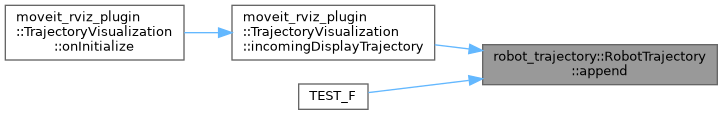## ◆ begin()

 RobotTrajectory::Iterator robot_trajectory::RobotTrajectory::begin ( )
inline

Definition at line 360 of file robot_trajectory.h.

## ◆ clear()

 RobotTrajectory& robot_trajectory::RobotTrajectory::clear ( )
inline

Definition at line 249 of file robot_trajectory.h.

Here is the caller graph for this function:## ◆ empty()

 bool robot_trajectory::RobotTrajectory::empty ( ) const
inline

Definition at line 180 of file robot_trajectory.h.

Here is the caller graph for this function: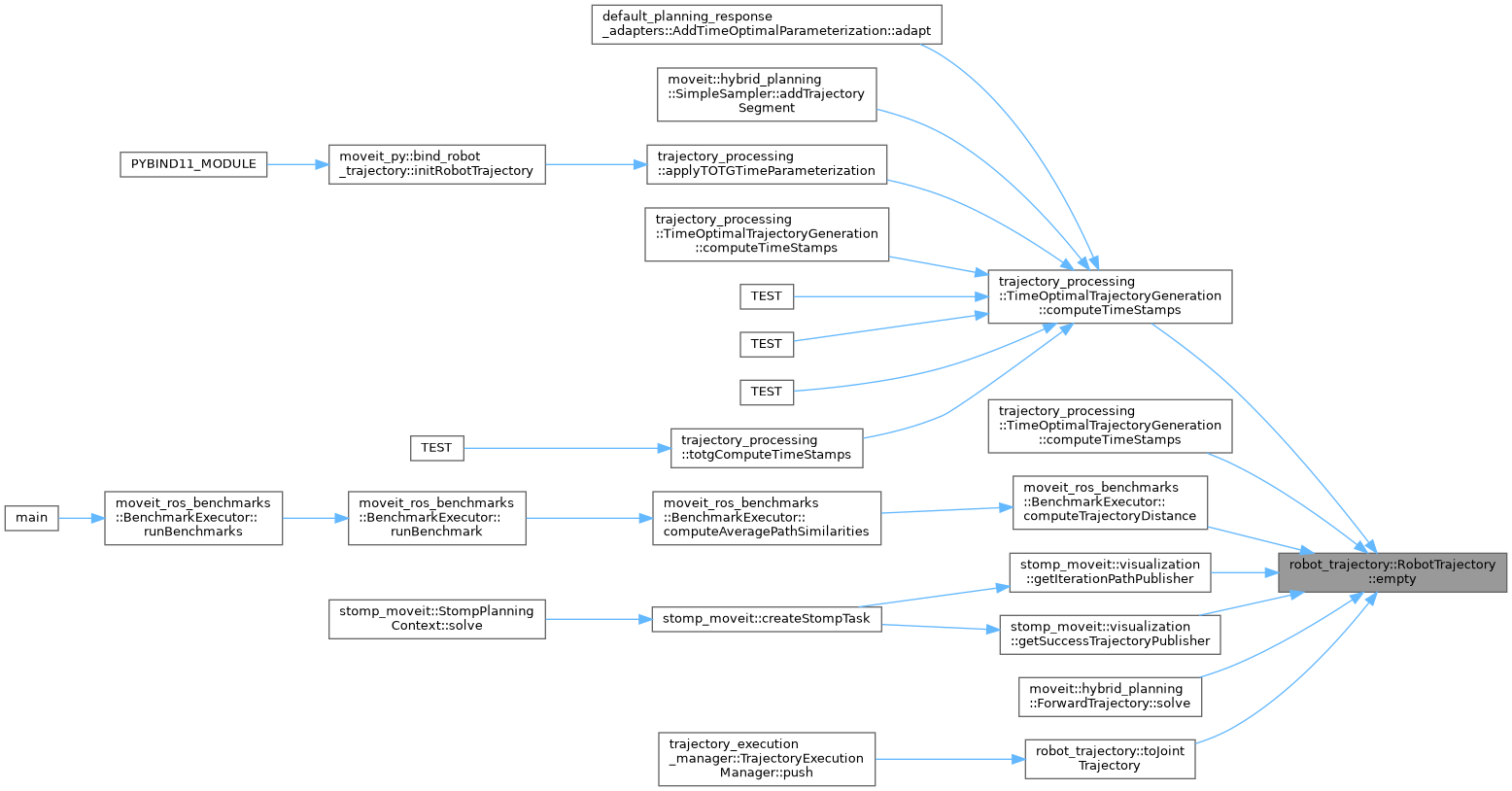## ◆ end()

 RobotTrajectory::Iterator robot_trajectory::RobotTrajectory::end ( )
inline

Definition at line 365 of file robot_trajectory.h.

## ◆ findWayPointIndicesForDurationAfterStart()

 void robot_trajectory::RobotTrajectory::findWayPointIndicesForDurationAfterStart ( const double & duration, int & before, int & after, double & blend ) const

Finds the waypoint indices before and after a duration from start.

Parameters
 The duration from start. The waypoint index before the supplied duration. The waypoint index after (or equal to) the supplied duration. The progress (0 to 1) between the two waypoints, based on time (not based on joint distances).

Definition at line 493 of file robot_trajectory.cpp.

Here is the caller graph for this function:## ◆ getAverageSegmentDuration()

 double robot_trajectory::RobotTrajectory::getAverageSegmentDuration ( ) const

Definition at line 92 of file robot_trajectory.cpp.

Here is the call graph for this function: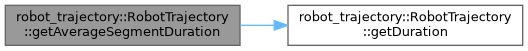Here is the caller graph for this function: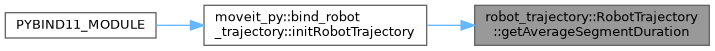## ◆ getDuration()

 double robot_trajectory::RobotTrajectory::getDuration ( ) const

Definition at line 87 of file robot_trajectory.cpp.

Here is the caller graph for this function: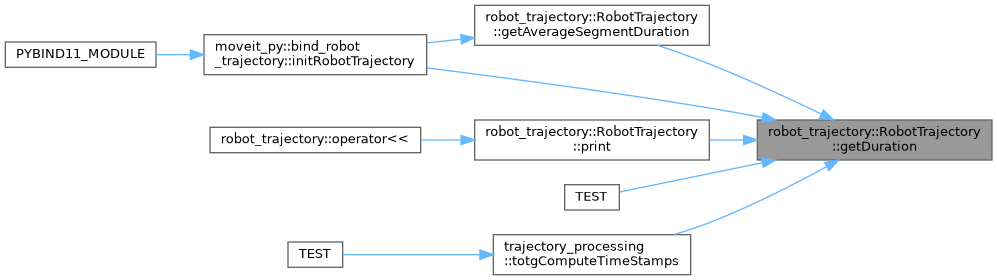## ◆ getFirstWayPoint()

 const moveit::core::RobotState& robot_trajectory::RobotTrajectory::getFirstWayPoint ( ) const
inline

Definition at line 127 of file robot_trajectory.h.

## ◆ getFirstWayPointPtr()

 moveit::core::RobotStatePtr& robot_trajectory::RobotTrajectory::getFirstWayPointPtr ( )
inline

Definition at line 142 of file robot_trajectory.h.

Here is the caller graph for this function:## ◆ getGroup()

 const moveit::core::JointModelGroup* robot_trajectory::RobotTrajectory::getGroup ( ) const
inline

Definition at line 93 of file robot_trajectory.h.

Here is the caller graph for this function:## ◆ getGroupName()

 const std::string & robot_trajectory::RobotTrajectory::getGroupName ( ) const

Definition at line 79 of file robot_trajectory.cpp.

Here is the call graph for this function: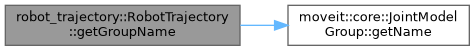Here is the caller graph for this function: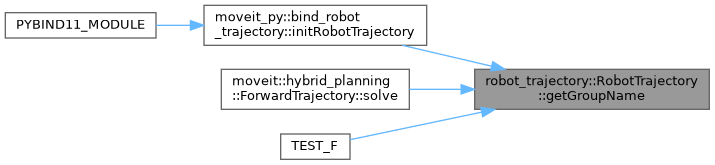## ◆ getLastWayPoint()

 const moveit::core::RobotState& robot_trajectory::RobotTrajectory::getLastWayPoint ( ) const
inline

Definition at line 122 of file robot_trajectory.h.

Here is the caller graph for this function:## ◆ getLastWayPointPtr()

 moveit::core::RobotStatePtr& robot_trajectory::RobotTrajectory::getLastWayPointPtr ( )
inline

Definition at line 137 of file robot_trajectory.h.

## ◆ getRobotModel()

 const moveit::core::RobotModelConstPtr& robot_trajectory::RobotTrajectory::getRobotModel ( ) const
inline

Definition at line 88 of file robot_trajectory.h.

Here is the caller graph for this function:## ◆ getRobotTrajectoryMsg()

 void robot_trajectory::RobotTrajectory::getRobotTrajectoryMsg ( moveit_msgs::msg::RobotTrajectory & trajectory, const std::vector< std::string > & joint_filter = `std::vector()` ) const

Definition at line 256 of file robot_trajectory.cpp.

Here is the call graph for this function: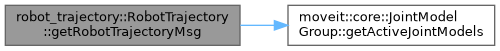Here is the caller graph for this function: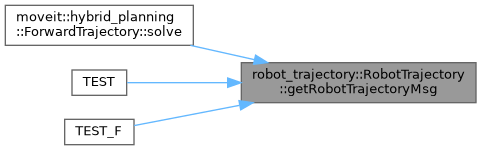## ◆ getStateAtDurationFromStart()

 bool robot_trajectory::RobotTrajectory::getStateAtDurationFromStart ( const double request_duration, moveit::core::RobotStatePtr & output_state ) const

Gets a robot state corresponding to a supplied duration from start for the trajectory, using linear time interpolation.

Parameters
 The duration from start. The resulting robot state.
Returns
True if state is valid, false otherwise (trajectory is empty).

Definition at line 546 of file robot_trajectory.cpp.

Here is the call graph for this function: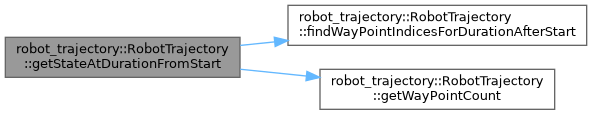## ◆ getWayPoint()

 const moveit::core::RobotState& robot_trajectory::RobotTrajectory::getWayPoint ( std::size_t index ) const
inline

Definition at line 117 of file robot_trajectory.h.

Here is the caller graph for this function:## ◆ getWayPointCount()

 std::size_t robot_trajectory::RobotTrajectory::getWayPointCount ( ) const
inline

Definition at line 106 of file robot_trajectory.h.

Here is the caller graph for this function: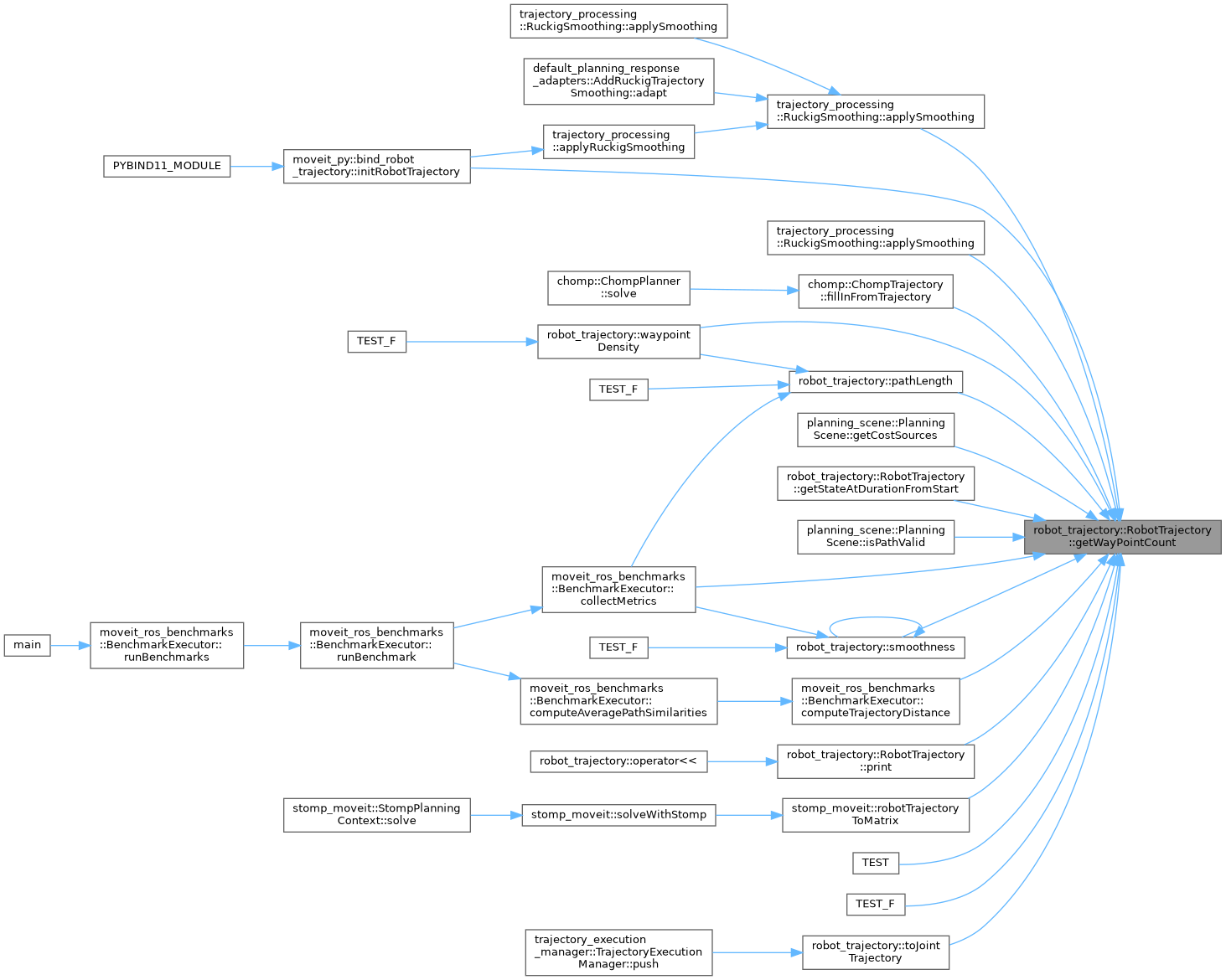## ◆ getWayPointDurationFromPrevious()

 double robot_trajectory::RobotTrajectory::getWayPointDurationFromPrevious ( std::size_t index ) const
inline

Definition at line 160 of file robot_trajectory.h.

Here is the caller graph for this function: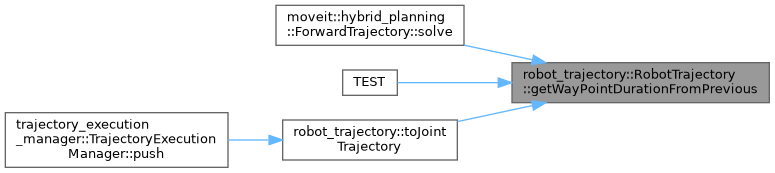## ◆ getWayPointDurationFromStart()

 double robot_trajectory::RobotTrajectory::getWayPointDurationFromStart ( std::size_t index ) const

Returns the duration after start that a waypoint will be reached.

Parameters
 The waypoint index.
Returns
The duration from start; returns overall duration if index is out of range.

Definition at line 528 of file robot_trajectory.cpp.

Here is the caller graph for this function: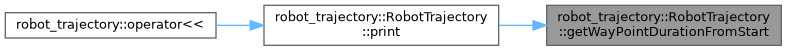## ◆ getWaypointDurationFromStart()

 double robot_trajectory::RobotTrajectory::getWaypointDurationFromStart ( std::size_t index ) const

Definition at line 541 of file robot_trajectory.cpp.

Here is the call graph for this function:## ◆ getWayPointDurations()

 const std::deque& robot_trajectory::RobotTrajectory::getWayPointDurations ( ) const
inline

Definition at line 147 of file robot_trajectory.h.

Here is the caller graph for this function:## ◆ getWayPointPtr()

 moveit::core::RobotStatePtr& robot_trajectory::RobotTrajectory::getWayPointPtr ( std::size_t index )
inline

Definition at line 132 of file robot_trajectory.h.

## ◆ insertWayPoint() [1/2]

 RobotTrajectory& robot_trajectory::RobotTrajectory::insertWayPoint ( std::size_t index, const moveit::core::RobotState & state, double dt )
inline

Definition at line 221 of file robot_trajectory.h.

Here is the caller graph for this function:## ◆ insertWayPoint() [2/2]

 RobotTrajectory& robot_trajectory::RobotTrajectory::insertWayPoint ( std::size_t index, const moveit::core::RobotStatePtr & state, double dt )
inline

Definition at line 226 of file robot_trajectory.h.

## ◆ operator=()

 RobotTrajectory& robot_trajectory::RobotTrajectory::operator= ( const RobotTrajectory & )
default

Assignment operator, performing a shallow copy, i.e. copying waypoints by pointer

## ◆ print()

 void robot_trajectory::RobotTrajectory::print ( std::ostream & out, std::vector< int > variable_indexes = `std::vector()` ) const

Parameters
 out Stream to print to variable_indexes The indexes of the variables to print. If empty/not specified and the group is defined, then uses the indexes for the group If empty and the group is not defined, uses ALL variables in robot_model

e.g. Trajectory has 13 points over 2.965 seconds waypoint 0 time 0.000 pos 0.000 vel 0.000 acc 0.000 waypoint 1 time 0.067 pos 0.001 vel 0.033 acc 1.000 waypoint 2 time 0.665 pos 0.200 vel 0.632 acc 1.000 ...

Definition at line 562 of file robot_trajectory.cpp.

Here is the call graph for this function: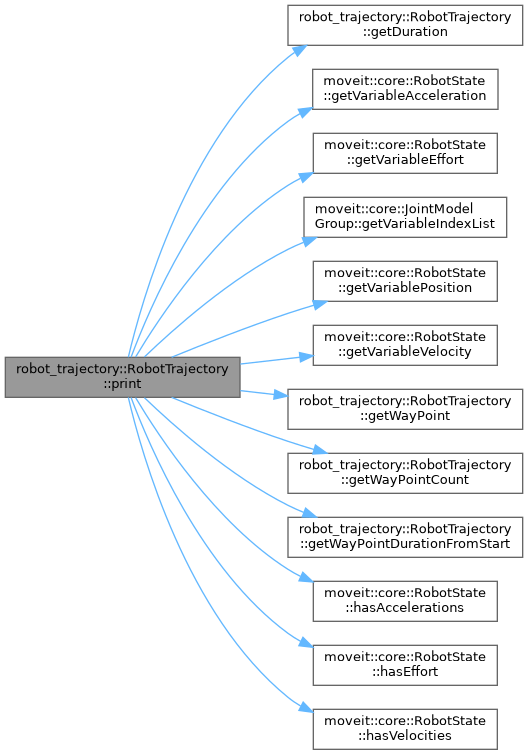Here is the caller graph for this function: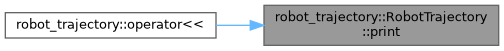## ◆ reverse()

 RobotTrajectory & robot_trajectory::RobotTrajectory::reverse ( )

Definition at line 140 of file robot_trajectory.cpp.

Here is the caller graph for this function: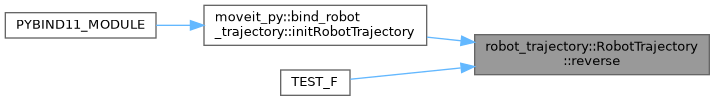## ◆ setGroupName()

 RobotTrajectory& robot_trajectory::RobotTrajectory::setGroupName ( const std::string & group_name )
inline

Definition at line 100 of file robot_trajectory.h.

Here is the caller graph for this function:## ◆ setRobotTrajectoryMsg() [1/3]

 RobotTrajectory & robot_trajectory::RobotTrajectory::setRobotTrajectoryMsg ( const moveit::core::RobotState & reference_state, const moveit_msgs::msg::RobotState & state, const moveit_msgs::msg::RobotTrajectory & trajectory )

Copy the content of the trajectory message into this class. The trajectory message itself is not required to contain the values for all joints. For this reason a full starting state must be specified as reference (reference_state). Before use, the reference state is updated using state. Each point in the trajectory to be constructed internally is obtained by copying the reference state and overwriting the content from a trajectory point in trajectory.

Definition at line 484 of file robot_trajectory.cpp.

Here is the call graph for this function: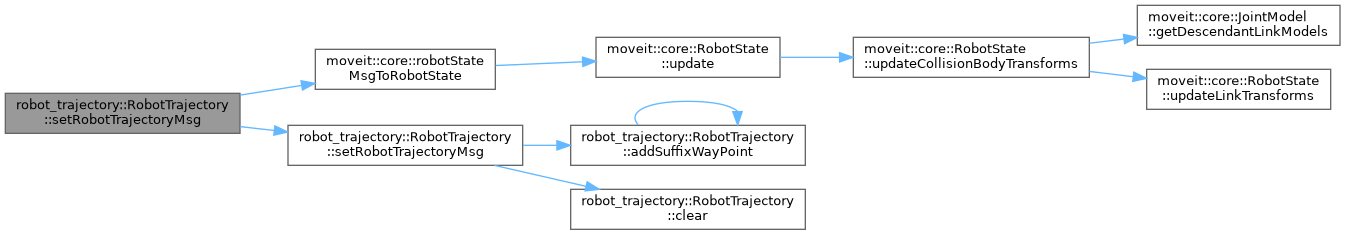## ◆ setRobotTrajectoryMsg() [2/3]

 RobotTrajectory & robot_trajectory::RobotTrajectory::setRobotTrajectoryMsg ( const moveit::core::RobotState & reference_state, const moveit_msgs::msg::RobotTrajectory & trajectory )

Copy the content of the trajectory message into this class. The trajectory message itself is not required to contain the values for all joints. For this reason a full starting state must be specified as reference (reference_state). Each point in the trajectory to be constructed internally is obtained by copying the reference state and overwriting the content from a trajectory point in trajectory.

Definition at line 432 of file robot_trajectory.cpp.

Here is the call graph for this function: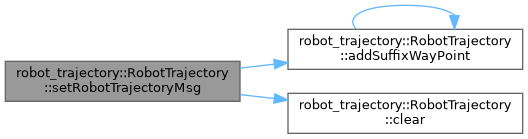## ◆ setRobotTrajectoryMsg() [3/3]

 RobotTrajectory & robot_trajectory::RobotTrajectory::setRobotTrajectoryMsg ( const moveit::core::RobotState & reference_state, const trajectory_msgs::msg::JointTrajectory & trajectory )

Copy the content of the trajectory message into this class. The trajectory message itself is not required to contain the values for all joints. For this reason a full starting state must be specified as reference (reference_state). Each point in the trajectory to be constructed internally is obtained by copying the reference state and overwriting the content from a trajectory point in trajectory.

Definition at line 404 of file robot_trajectory.cpp.

Here is the call graph for this function:Here is the caller graph for this function: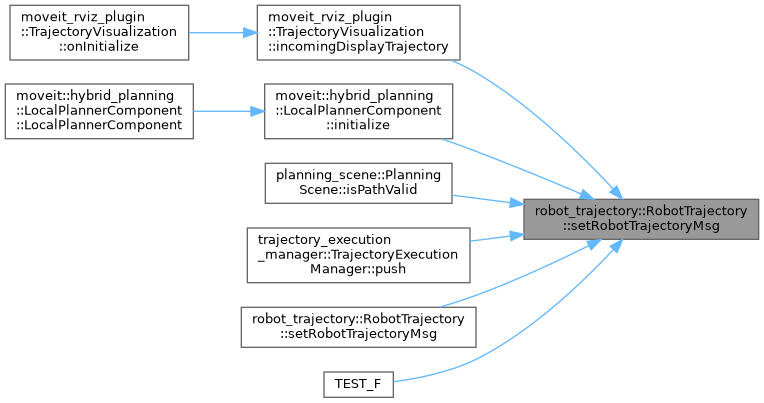## ◆ setWayPointDurationFromPrevious()

 RobotTrajectory& robot_trajectory::RobotTrajectory::setWayPointDurationFromPrevious ( std::size_t index, double value )
inline

Definition at line 172 of file robot_trajectory.h.

## ◆ size()

 std::size_t robot_trajectory::RobotTrajectory::size ( ) const
inline

Definition at line 111 of file robot_trajectory.h.

## ◆ swap()

 void robot_trajectory::RobotTrajectory::swap ( robot_trajectory::RobotTrajectory & other )

Definition at line 115 of file robot_trajectory.cpp.

Here is the caller graph for this function:## ◆ unwind() [1/2]

 RobotTrajectory & robot_trajectory::RobotTrajectory::unwind ( )

Definition at line 158 of file robot_trajectory.cpp.

Here is the call graph for this function: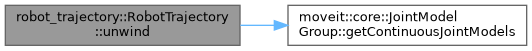Here is the caller graph for this function: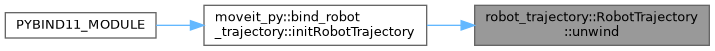## ◆ unwind() [2/2]

 RobotTrajectory & robot_trajectory::RobotTrajectory::unwind ( const moveit::core::RobotState & state )

Unwind, starting from an initial state.

Definition at line 200 of file robot_trajectory.cpp.

Here is the call graph for this function: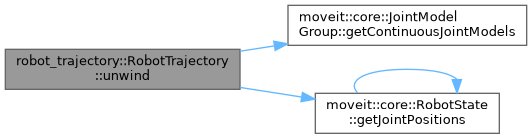The documentation for this class was generated from the following files: